Start typing, then use the up and down arrows to select an option from the list.# Endothermic & Exothermic Reactions

Jules Bruno
433views
1
1
Now Endo thermic reactions involve absorbing thermal energy by the system from the surroundings. And we're gonna say here as a result of absorbing this thermal energy, the molecules will start to speed up and if they're given enough energy, they can use it to break their bonds. So endo thermic reactions are heat absorbing bond breaking reactions here, we take a look if we look at it in terms of phase changes. If you're absorbing heat, it helps to spread molecules apart. Think about you have a solid here, the solid absorbs enough heat, thermal energy. Think of it as an ice cube. What happens to the ice cube? Over time? It melts. Now it's a liquid. Let's keep adding a little bit more heat to that liquid water. What happens to it eventually it vaporizes into a gas here, We're breaking the connections between water molecules as it goes from solid liquid to gas. Now, if you're going from a solid to a liquid that is melting or what we call fusion. In terms of thermal chemistry, if you're going from a liquid to a gas that's vaporization and then if you're going from a solid to a gas that's called sublimation. Now, real world applications, if you are an endo thermic reaction, you're absorbing heat from the surroundings. Now, if I were to touch an endo thermic reaction that's contained within a container, what would it do? Well, it would absorb heat from my hand. So the the substance is absorbing heat from my hand. So it feels cold to me because I'm losing heat from my hand to the container. Finally, in terms of an energy diagram, energy diagrams our way of showing how a reaction progresses. In terms of energy, you start off as a reactant to get to your final product as your last stop. In an endo thermic reaction, your entropy value, which is delta H, is positive, it's a positive sign here. The beginning part of this energy diagram is represented as our reactant and then here's where you end. This represents our products, here are y axis is energy. So in an endo thermic reaction are react and start off at a lower energy and our products and at a higher energy. This gives us a positive entropy or delta H.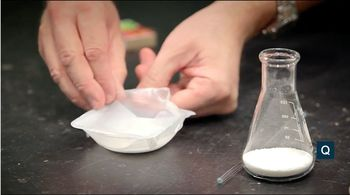07:23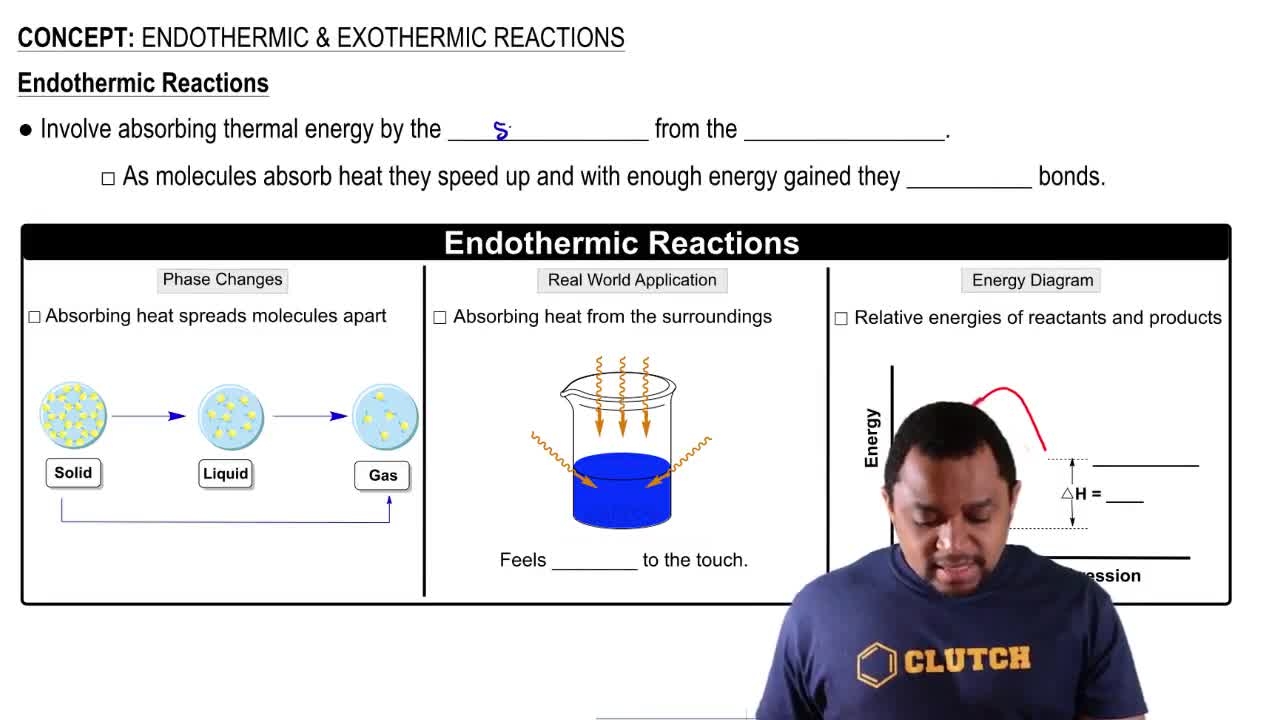02:30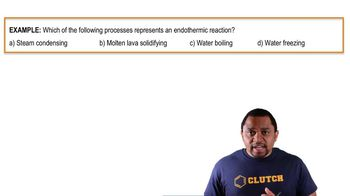00:55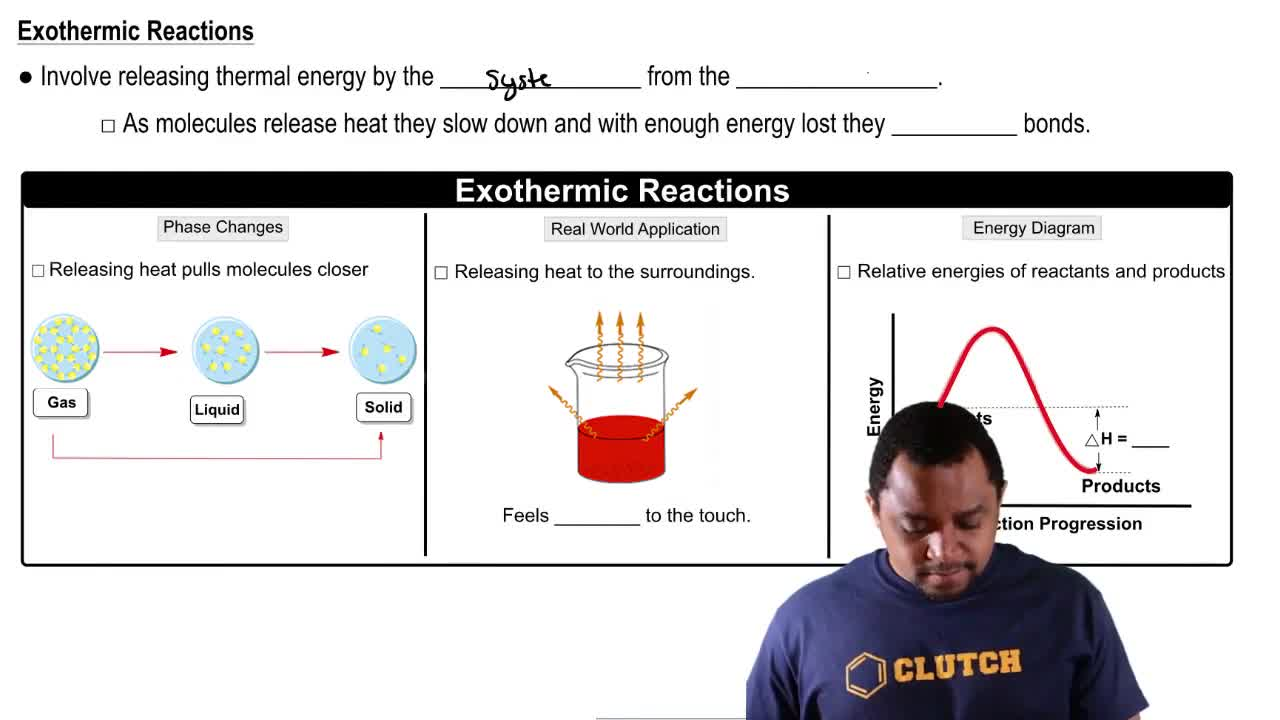02:38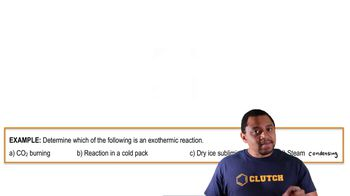01:23